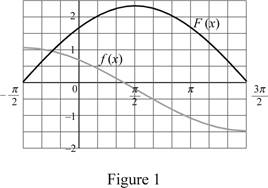# The value of the indefinite integral function ∫ cos x 1 + sin x d x .### Single Variable Calculus: Concepts...

4th Edition
James Stewart
Publisher: Cengage Learning
ISBN: 9781337687805### Single Variable Calculus: Concepts...

4th Edition
James Stewart
Publisher: Cengage Learning
ISBN: 9781337687805

#### Solutions

Chapter 5, Problem 35RE
To determine

## The value of the indefinite integral function ∫cosx1+sinxdx.

Expert Solution

The value of the indefinite integral is 21+sinx+C_

### Explanation of Solution

Given information:

The integral function is cosx1+sinxdx.

Consider (1+sinx) as u.

u=1+sinx (1)

Differentiate both sides of the Equation (1).

du=cosxdx (2)

Substitute u for (1+sinx) and du for cosxdx in the function as shown below.

cosx1+sinxdx=1udu (3)

The expression to find the indefinite integral value using Equation (3) as shown below.

cosx1+sinxdx=1udu=1u12du=u12du=(u12+112+1)

=(u12+112+1)=u1212=2u12 (4)

Substitute 1+sinx for u in Equation (1) as shown below.

cosx1+sinxdx=2(1+sinx)1/2=21+sinx+C

Therefore, the value of the indefinite integral is 21+sinx+C_

Apply the Theorem:

The integral function f(x)dx is traditionally used for an antiderivative of f and is called an indefinite integral.

f(x)dx=F(x) (5)

Substitute cosx1+sinx for f(x) and 21+sinx+C for F(x) in Equation (5) as shown below.

cosx1+sinxdx=21+sinx+C (6)

Draw the graph for the function (f(x)) and its antiderivative (F(x)) using Equation (6) as shown below.Refer to Figure 1.

The function F(x) Increases with positive value of f(x) and F(x) decreases with negative value of f(x).

The minimum value of the function occurs at f(x)=0. Hence, it is reasonable from the graphical evidence.

Therefore, F(x) is an antiderivative of f(x).

### Have a homework question?

Subscribe to bartleby learn! Ask subject matter experts 30 homework questions each month. Plus, you’ll have access to millions of step-by-step textbook answers!